# Planimetrics - math word problems

Study plane measurements, including angles, distances, and areas. In other words - measurement and calculation of shapes in the plane. Perimeter and area of plane shapes.

#### Number of problems found: 1947

• Wall diagonalCalculate the length of wall diagonal of the cube whose surface is 384 cm square.
• Diameter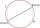If the endpoints of a diameter of a circle are A(10, -1) and B (3, 10), what is the radius of the circle?
• Chord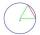Point on the circle is the end point of diameter and end point of chord length of radius. What angle between chord and diameter?
• Triangle anglesThe angles α, β, γ in triangle ABC are in the ratio 6:2:6. Calculate size of angles.
• Clock Tower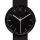What angle is betwenn hands on Clock Tower when show 17 hours and 35 minutes?
• RT sidesFind the sides of a rectangular triangle if legs a + b = 17cm and the radius of the written circle ρ = 2cm.
• Axial cutThe cone surface is 388.84 cm2, the axial cut is an equilateral triangle. Find the cone volume.
• Smallest internal angle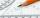Calculate what size has the smallest internal angle of the triangle if values of angles α:β:γ = 3:4:8
• Garden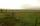The garden has two opposite parallel fences. Their distance is 33.1 m. Lengths in these two fences are 75.5 meters and 49.4 meters. Calculate the area of this garden.
• Cellar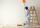Cellar for storing fruit has a rectangular base with sides 14 m and 7 meters. You should paint sidewall to 2 m. How m2 surface must be painted?
• Medians and sides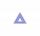Determine the size of a triangle KLM and the size of the medians in the triangle. K=(-5; -6), L=(7; -2), M=(5; 6).
• Isosceles trapeziumCalculate the area of an isosceles trapezium ABCD if a = 10cm, b = 5cm, c = 4cm.
• Arc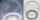The length of the circle is 41 amd arc length of the circle 9. What is the magnitude of the angle of this arc?
• Coordinates of a centroindLet’s A = [3, 2, 0], B = [1, -2, 4] and C = [1, 1, 1] be 3 points in space. Calculate the coordinates of the centroid of △ABC (the intersection of the medians).
• Trapezoid - RRFind the area of the right angled trapezoid ABCD with the right angle at the A vertex; a = 3 dm b = 5 dm c = 6 dm d = 4 dm
• The Eiffel Tower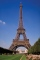The top of the Eiffel Tower is seen from a distance of 600 meters at an angle of 30 degrees. Find the tower height.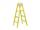The double ladder has 3 meters long shoulders. What is the height of the upper of the ladder reach if the lower ends are 1.8 meters apart?
• The bridge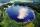Across the circle lakepasses through its center bridge over the lake. At three different locations on the lake shore are three fishermen A, B, C. Which of fishermen see the bridge under the largest angle?
• Tiles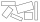The tile has the shape of a square with a side of 15 cm. What dimensions can a rectangle composed of 90 of these tiles have so that no tile remains?How long is a ladder that touches on a wall 4 meters high and its lower part is 3 meters away from the wall?